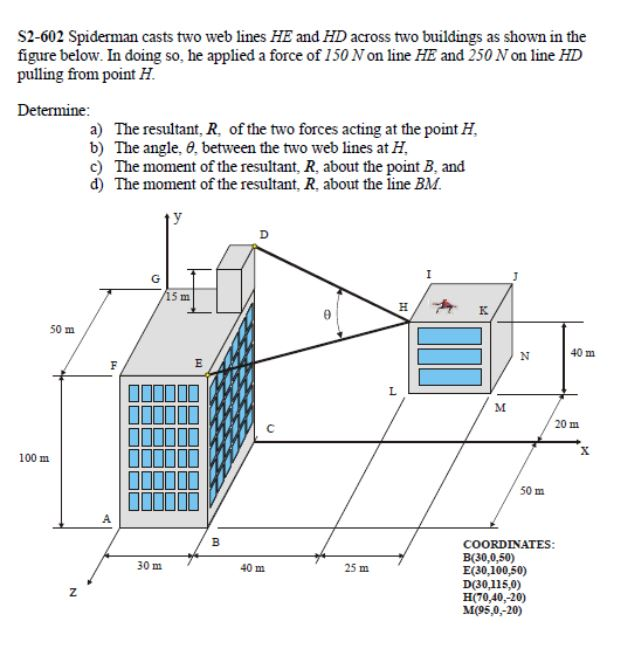# S2-602 Spiderman casts two web lines HE and HD across two buildings as shown in the...

###### Question:S2-602 Spiderman casts two web lines HE and HD across two buildings as shown in the figure below. In doing so, he applied a force of 150 N on line HE and 250 N on line HD pulling from point H Determine: a) The resultant, R, of the two forces acting at the point H b) The angle, , between the two web lines at H c) The moment of the resultant, R, about the point B, and d) The moment of the resultant, R, about the line BM y 15 m H K 50 m 40 m N E L M 20 m C X 100 m 50 m A COORDINATES: B(30,0,50) E(30,100,50) D(30,115,0) H(70,40,20) M05,0-20) 30 m 40 m 25 m

#### Similar Solved Questions

##### Solve questions 2-5 completely For each set of values, determine whether an exponential function is a good model. I...
solve questions 2-5 completely For each set of values, determine whether an exponential function is a good model. If so, find the function. If not, explain why (2 pts) 9 y 6 22 54 102 145 The exponential function isn't the best model since the values from the model is farther than actual val...
##### Estion 18 and Two-dimensional gel electrophoresis separates proteins based on a. size; shape b.shape; charge C....
estion 18 and Two-dimensional gel electrophoresis separates proteins based on a. size; shape b.shape; charge C. size; concentration d. size; charge e concentration; shape Question 14 When eukaryotic genomes and prokaryotic genomes are compared, a. in eukaryotic genomes, there is a single origin of r...
##### Me Question2: why does benzoylation not happen twice on the same ringe ferro cenewt 1at Question3:...
me Question2: why does benzoylation not happen twice on the same ringe ferro cenewt 1at Question3: Calculate the volume of 3 M aqueous sodium hydroxide needed to neutralize the acetic acid and phosphoric acids in the synthesis of acetylferrocene. Question4: What feature of the benzoylation of fer...